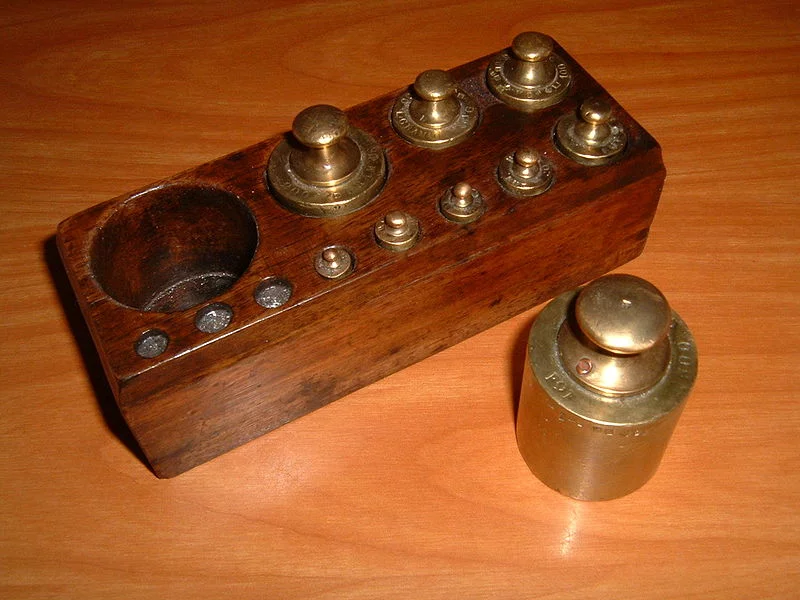## Linear Combination

### DefinitionsA linear combination in mathematics is an expression constructed from a set of terms by multiplying each term by a constant and adding the results.

Example of a linear combination:
a · x + b · y is a linear combination of x and y with a and b constants.

Generally;
p = λ1 · x1 + λ2 · x2 … λn · xn
p is the scalar product of the values x1, x2 … xn and
λ1, λ2 … λn are called scalars.
In most applications x1, x2 … xn are vectors and the lambdas are integers or real numbers. (For those, who prefer it mor formally: x1, x2 … xn ∈ V and V is a vector space, and
λ1, λ2 … λn ∈ K with K being a field)

</p>

### Linear Combinations in Python

The vector y = (3.21, 1.77, 3.65) can be easily written as a linear combination of the unit vectors (0,0,1), (0,1,0) and (1,0,0):

(3.21, 1.77, 3.65) = 3.21 · (1,0,0) + 1.77 (0,1,0) + 3.65 · (0,0,1)

We can do the calculation with Python, using the module numpy:

import numpy as np
x = np.array([[0, 0, 1],
[0, 1, 0],
[1, 0, 0]])
y = ([3.65, 1.55, 3.42])
scalars = np.linalg.solve(x, y)
scalars

Output:
array([3.42, 1.55, 3.65])

The previous example was very easy, because we could work out the result in our head. What about writing our vector y = (3.21, 1.77, 3.65) as a linear combination of the vectors (0,1,1), (1,1,0) and (1,0,1)? It looks like this in Python:

import numpy as np
x = np.array([[0, 1, 1],
[1, 1, 0],
[1, 0, 1]])
y = ([3.65, 1.55, 3.42])
scalars = np.linalg.solve(x, y)
scalars

Output:
array([0.66, 0.89, 2.76])

### Another Example

Any integer between -40 and 40 can be written as a linear combination of 1, 3, 9, 27 with scalars being elements of the set {-1, 0, 1}.
For example:
7 = 1 · 1 + (-1) · 3 + 1 · 9 + 0 · 27

We can calculate these scalars with Python. First we need a generator generating all the possible scalar combinations. If you have problems in understanding the concept of a generator, we recommend the chapter "Iterators and Generators" of our tutorial.

def factors_set():
for i in [-1, 0, 1]:
for j in [-1,0,1]:
for k in [-1,0,1]:
for l in [-1,0,1]:
yield (i, j, k, l)


We will use the memoize() technique (see chapter "Memoization and Decorators" of our tutorial) to memorize previous results:

def memoize(f):
results = {}
def helper(n):
if n not in results:
results[n] = f(n)
return results[n]
return helper


Finally, in our function linear_combination() we check every scalar tuple, if it can create the value n:

@memoize
def linear_combination(n):
""" returns the tuple (i,j,k,l) satisfying
n = i*1 + j*3 + k*9 + l*27      """
weighs = (1,3,9,27)

for factors in factors_set():
sum = 0
for i in range(len(factors)):
sum += factors[i] * weighs[i]
if sum == n:
return factors


Putting it all together results in the following script:

def factors_set():
for i in [-1, 0, 1]:
for j in [-1, 0, 1]:
for k in [-1, 0, 1]:
for l in [-1, 0, 1]:
yield (i, j, k, l)

def memoize(f):
results = {}
def helper(n):
if n not in results:
results[n] = f(n)
return results[n]
return helper

@memoize
def linear_combination(n):
""" returns the tuple (i,j,k,l) satisfying
n = i*1 + j*3 + k*9 + l*27      """
weighs = (1, 3, 9, 27)

for factors in factors_set():
sum = 0
for i in range(len(factors)):
sum += factors[i] * weighs[i]
if sum == n:
return factors

# calculate the linear combinations of the first 10 positive integers:
for i in range(1,11):
print(linear_combination(i))

(1, 0, 0, 0)
(-1, 1, 0, 0)
(0, 1, 0, 0)
(1, 1, 0, 0)
(-1, -1, 1, 0)
(0, -1, 1, 0)
(1, -1, 1, 0)
(-1, 0, 1, 0)
(0, 0, 1, 0)
(1, 0, 1, 0)